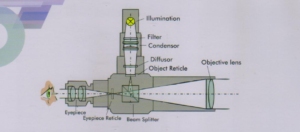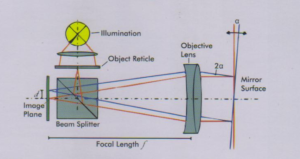# Autocollimator Model KW-950 Auto

## Description

Model KW-950

ALMICRO AUTOCOLLIMATOR is a sensitive, extremely accurate optical instrument which is precisely used in optical workshops, inspection departments and quality control labs.
It is basically a comparator where we compare optical element like slabs and prisms for flatness. It is used for very small angle measurements of the   order of minutes.The Autocollimator combines both optical tools, the collimator and the telescope into one instrument using a single objective lens. Both beam paths are separated by using a beam splitter The autocollimator is a very sensitive angle measuring device and is thus used for the precise angular adjustment of optical or machine components. Due to the collimated beam (infinity adjustment) the measurement results are independent from the distance to the object under test The operating principle is explained in the following.Operating Principle of an Autocollimator Like in the collimator the image of the illuminated object reticle is projected by the objective lens to infinity. In some distance, the collimated beam is reflected back from a mirrored surface. If the mirror surface is tilted by an angle a with respect to the optical axis, the reflected beam will enter the objective lens with an angle 2a. This leads to a shift d of the image in the image plane which can be calculated with the objective focal length f giving d = 2a x f or a = d/( 2f ). Thus, the sample angle is directly proportional to the measured shift in the image plane (small angles assumed). The resolution of an autocollimator increases proportionally and the angular field of view reciprocally with the focal length of the objective lens.

## Reviews

There are no reviews yet.